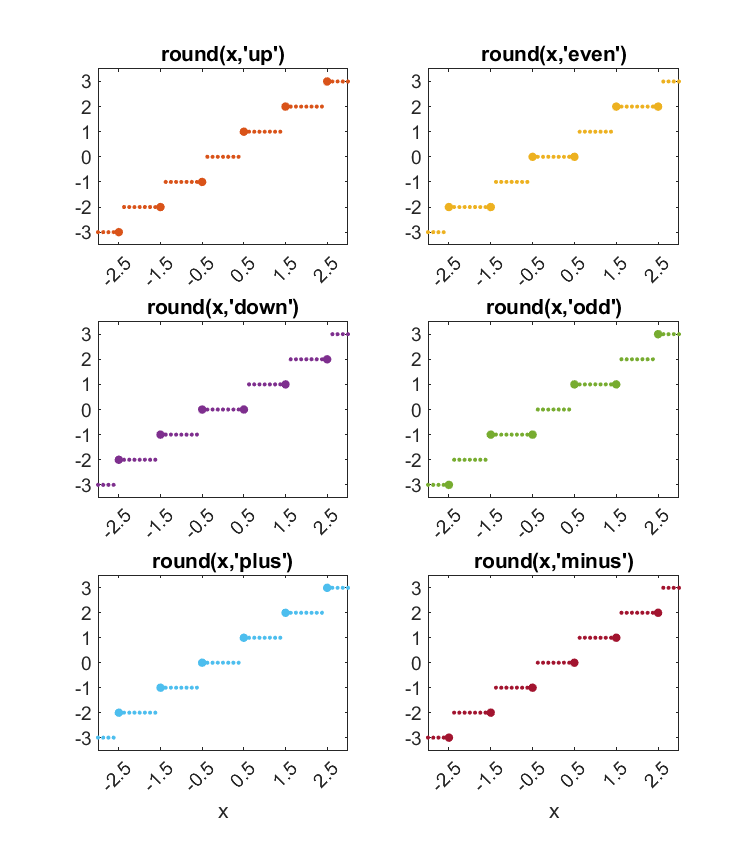# Round, With Tie Breakers, Round Two

I published Round, With Ties to Even a couple of days ago. Steve Eddins and Daniel Dolan immediately had substantive comments. Here is my reaction to their comments.

### Contents

#### Two More

The four choices that I described for how to break ties -- 'even', 'odd', 'up' and 'down' -- all have the same behavior for positive and negative numbers. The current built-in round uses the 'up" rule.

Steve sees the need for two more choices that are sensitive to the sign of the number. I will call them 'plus' and 'minus". They round to the right and to the left on the number line. That's sometimes known as round toward plus infinity and toward minus infinity.

#### Sample

Here's a chart of a few values.

    x = (-4.5:1:4.5)';
xRound(x);

     x     round  up  down   even   odd  plus minus

-4.500    -5    -5    -4    -4    -5    -4    -5
-3.500    -4    -4    -3    -4    -3    -3    -4
-2.500    -3    -3    -2    -2    -3    -2    -3
-1.500    -2    -2    -1    -2    -1    -1    -2
-0.500    -1    -1    -0    -0    -1    -0    -1
0.500     1     1     0     0     1     1     0
1.500     2     2     1     2     1     2     1
2.500     3     3     2     2     3     3     2
3.500     4     4     3     4     3     4     3
4.500     5     5     4     4     5     5     4


And the plot.#### Scaling

The current built-in round also includes the possibility of scaling by a power of 10 to facilitate rounding to, say, the nearest multiple of 1000, or of 1/1000, or three significant figures. Daniel was concerned that having both scaling and tie-breaking options in the same function would be confusing.

But, I think it is OK, because:

• round(x,'even'), the parameter 'even' is a char.
• round(x,3), the parameter 3 is numeric.
• round(x,3,'significant'), the parameter 'significant' is a specific char.

So, the statements

• round(x,'even',3)
• round(x,3,'even')
• round(x,'even',3,'significant')

are unambiguous and distinguishable. After x, the other parameters can be given in any order.

#### round

Here is my proposal for the enhancement request about round. It is available here.

   type round.m

function r = round(varargin)
% r = round(x) scales and rounds the elements of x to the nearest integers.
% Default: ties, elements halfway between integers, are rounded away from zero.
%
% r = round(x,'even') ties round to even integers.
% r = round(x,'odd') ties round to odd integers.
% r = round(x,'up') ties round away from zero (same as default).
% r = round(x,'down') ties round towards zero.
% r = round(x,'plus') ties round to the right on the number line.
% r = round(x,'minus') ties round to the left on the number line.
%
% r = round(x,n), n >= 0, round(10^n*x)/10^n, round(12.3456,2) = 12.3500
% r = round(x,-n), n > 0, 10^n*round(x/10^n), round(1234.56,-2) = 1200.
% r = round(x,n,'significant'), round(.001234,2,'significant') = .0012
% r = round(x,n,'decimals) same as round(x,n).
%
% r = round(x,...), ties, n, 'significant' and 'decimals' can be in any order.
%
% Use Round(...) with capital R to distinguish from built-in round(...).

[x,n,ties] = parse_input(varargin{:});
x = prescale(x,n);

a = abs(x) + 0.5;
r = floor(a);
switch ties
case 'even'
m = (r == a) & (mod(r,2) == 1);
case 'odd'
m = (r == a) & (mod(r,2) == 0);
case 'down'
m = (r == a);
case 'up'
m = [];
case 'plus'
m = (x < 0) & (r == a);
case 'minus'
m = (x > 0) & (r == a);
otherwise
error(['''' ties ''' not recognized.'])
end
r(m) = r(m) - 1;
r = sign(x).*r;

r = postscale(r,n);

% ----------------------------------------------

function [x,n,ties] = parse_input(varargin)
x = varargin{1};
n = zeros(size(x));
ties = 'up';
for k = 2:nargin
if isnumeric(varargin{k})
n(:) = varargin{k};
elseif strcmp(varargin{k},'significant')
n(:) = n(:) - ceil(log10(abs(x(:))));
elseif strcmp(varargin{k},'decimals')
% ignore
else
ties = varargin{k};
end
end
end

function x = prescale(x,n)
if any(n ~= 0)
k = n > 0;
x(k) = 10.^n(k).*x(k);
k = n < 0;
x(k) = x(k)./10.^(-n(k));
end
end

function r = postscale(r,n)
if any(n ~= 0)
k = n > 0;
r(k) = r(k)./10.^n(k);
k = n < 0;
r(k) = 10.^(-n(k)).*r(k);
end
end
end


Published with MATLAB® R2021a

|

### Comments

To leave a comment, please click here to sign in to your MathWorks Account or create a new one.